# How does a model predict the next word?

Exploring the way language models choose the next token. In here: Temperature, TopP, TopK

A language model knows how to complete texts token by token. But how does it choose the next token? Will it always choose the same token for a given sequence, or will it choose something different every time?

Given a prompt (the input to the model), the model generates a distribution over all possible completions (output from the model). It does that by calculating the probability of every token in the dictionary appearing next. Then, the model samples a completion according to the distribution.

Let's understand this by using a simple example. Suppose the prompt is the phrase "I will". Here is the distribution over all possible tokens (assuming a very small dictionary just for the demonstration):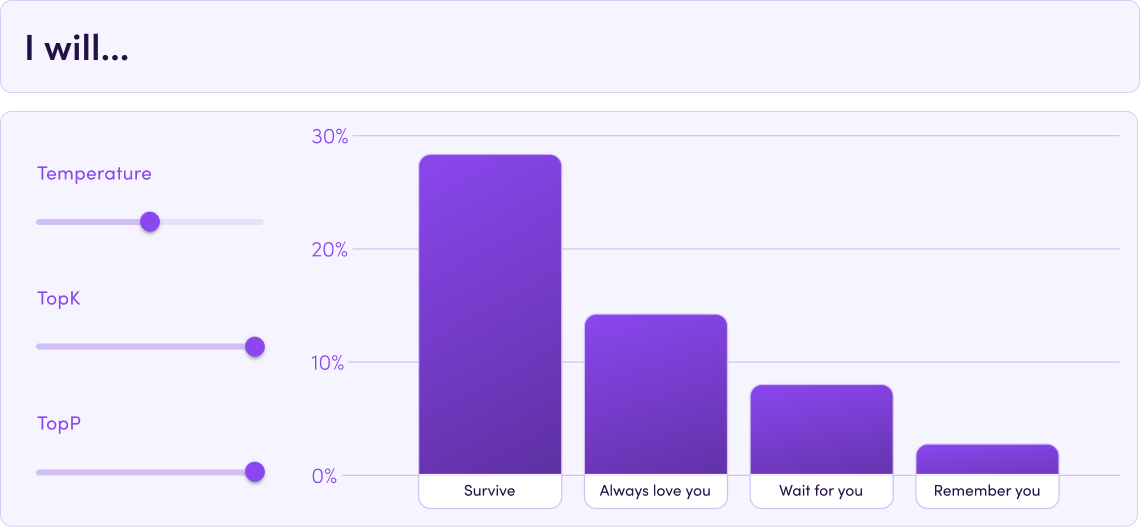There is a 55% chance that the model will choose the completion "survive", a 25% chance of the completion "always love you", etc. In simple words, it means that if we give the model this prompt 100 times, we'll likely get “survive” 55 times, "always love you" 25 times, etc.

As users, we want to have some control over the output, so we can get the most out of it. The key is to somehow change the distribution the model samples without changing anything in the model. Here are three ways to adjust the sampling of language models using sampling parameters. Each of them has its own benefits, and it is also possible to combine them. In the end, it all comes down to what you need.

## 🌡️ Temperature

The most popular sampling parameter to play with is the temperature. Inspired by thermodynamics, low temperature means stagnation and high temperature means movement. The temperature value affects the distribution: for a low temperature, we get a higher weight for the most probable token. At 0 temperature, we always get the maximum token, and the distribution looks like this: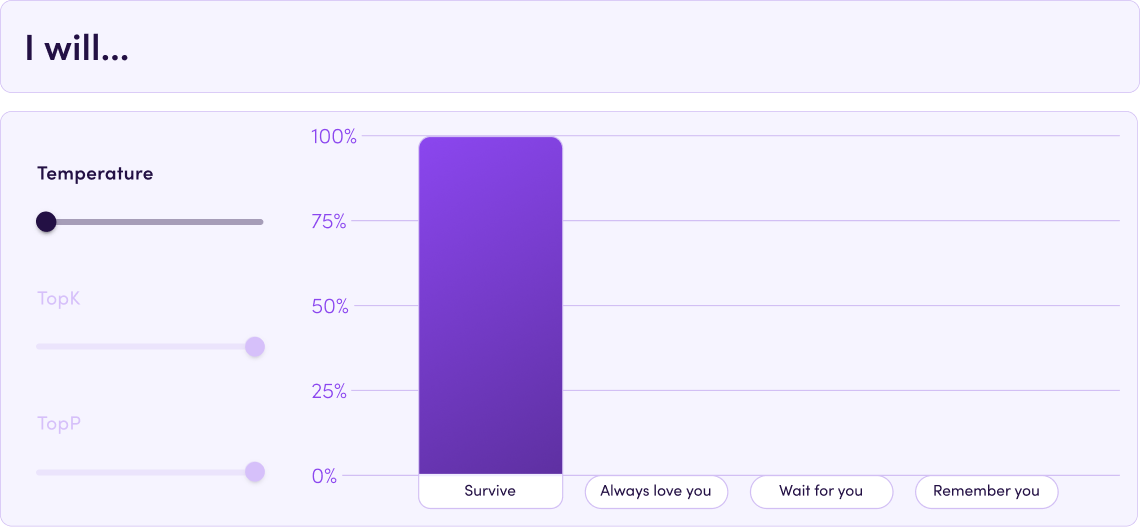Namely, the model will always sample the same token at every call.

A high temperature does the exact opposite: the distribution tends to be as uniform as possible, meaning all tokens have about the same probability. It will look something like this: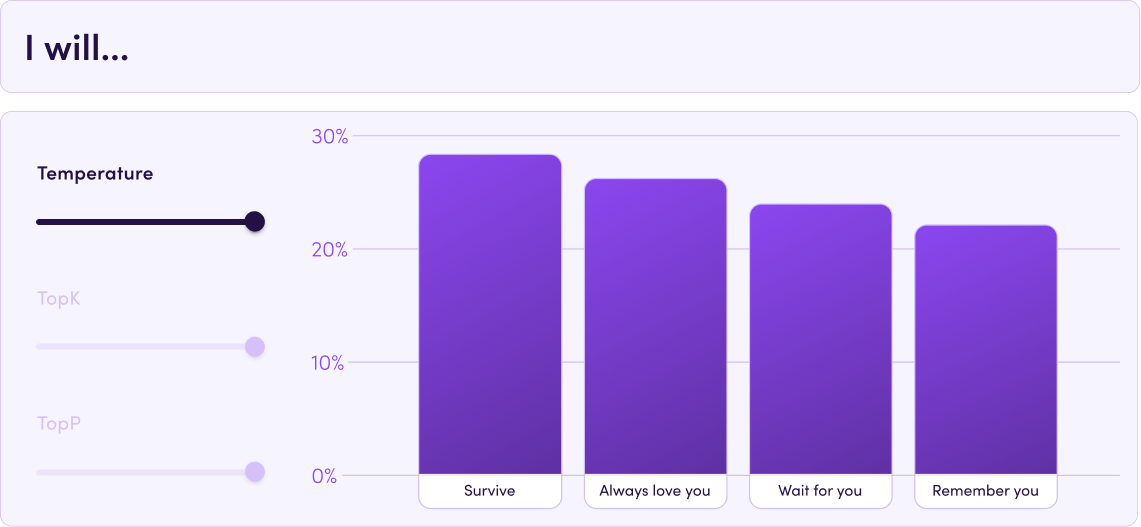So if you care about consistency and you always want to get the most likely completion (for example, in classification tasks), go for temperature=0. If you want more creativity (usually useful in text generation tasks), try a higher temperature (we recommend to start with 0.7).

## 📊 TopK

Another sampling parameter that helps with adjusting the model’s sampling is TopK. It’s pretty simple: instead of using the full distribution, the model only samples from the K most probable tokens. For K=1, we basically get the same situation as temperature=0. In our example, for K=2, the distribution looks like this: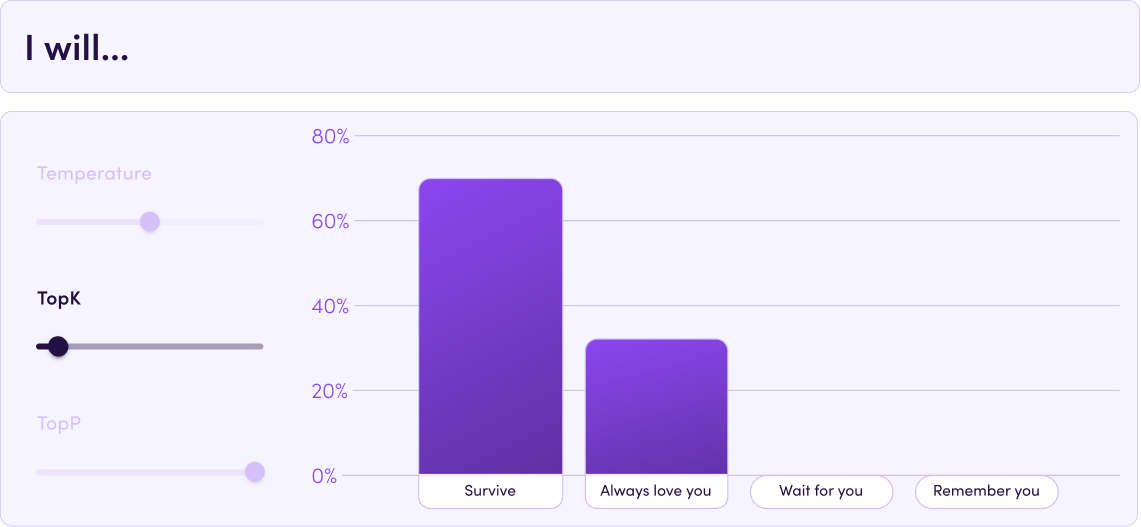## 📶 TopP

The third parameter is TopP. This is similar to TopK, but instead of limiting the number of tokens, we limit the total cumulative probability from which to sample. It works like this: we take the most likely token, add it to the pool, and add its probability to the amount. We continue adding tokens this way until the sum of probabilities reaches TopP.

What happens here is effectively similar to TopK: we remove the "tail" of the tokens with the lowest probabilities, except that TopP is more flexible for the number of tokens. In our example for TopP=0.95, we sample from the following distribution: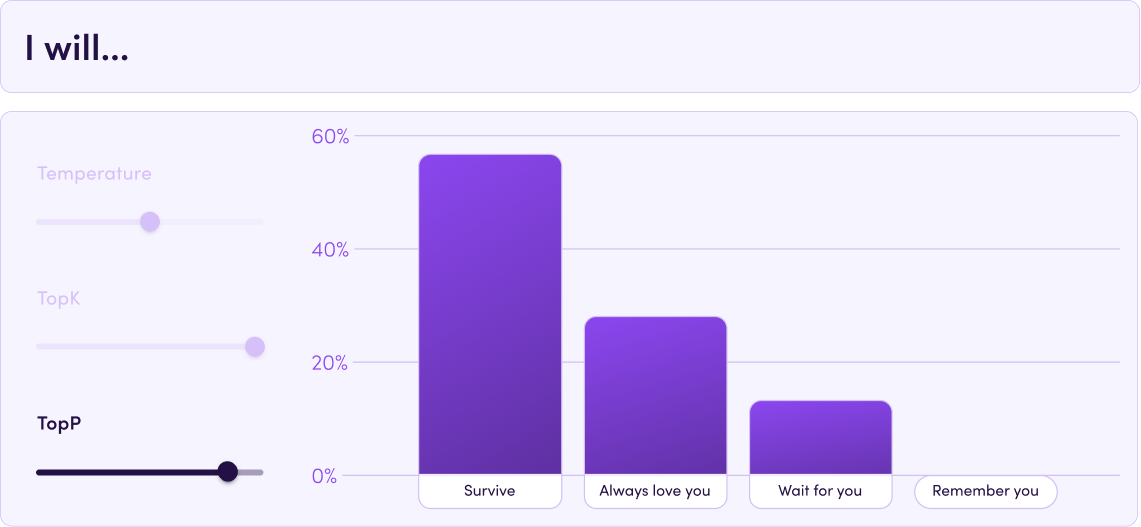## 🔨 What can I do with this information?

• If you want accuracy rather than creativity, go for temperature 0. This is useful in tasks of classification (Classification) or when extracting interesting details from texts (Extraction).
• If you deal with text generation tasks (whether short texts like tweets or long ones like whole posts) or getting ideas for slogans, for example, you probably need creativity. It is strongly recommended to raise the temp. Is the model getting a little too wacky? Keep the temperature high, but reduce the TopP/TopK and prepare to be amazed.